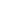科研动态最新资讯

• 天元讲堂（10.11）Qi Ye: Kernel-based Probability Measures for Data Analysis: A Deterministic or Stochastic Problem?
• 浏览量：110 发布人： 发布时间：2019-10-07
• 报告题目Kernel-based Probability Measures for Data Analysis: A Deterministic or Stochastic Problem?

报告人：Qi Ye华南师范大学

时间：2019年1014日（星期10:00—11:00

地点：苏州大学本部精正楼（数学楼）301

摘要In the talk, we introduce a concept of kernel-based probability measures on Banach spaces to solve a deterministic problem by a stochastic approach such as collocations and interpolations. We combine the theory of numerical analysis, regression analysis, and stochastic analysis to renew the classical kernel-based approximation methods for data analysis and machine learning. The kernel-based probability measures give a numerical tool to construct and analyze the kernel-based estimators based on the generalized data including kernel-based algorithms and error analysis.

欢迎参加！